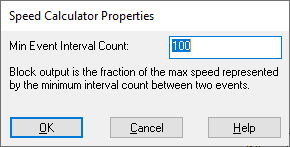## Speed Calculator

Embedded Category: C2407, F280x

Block Category: TI 16-bit DMC, TI 32-bit DMC

Operating Mode Availability

Simulation mode: YES

C code generation mode: YES

The Speed Calculator block calculates the motor speed based on an input time interval, such as the time between two successive events.

The Speed Calculator block is typically used in conjunction with an Event Capture block. The output of the Event Capture block, which represents the time interval between two successive events, is applied as the input to the Speed Calculator block.

The Speed Calculator block determines a 32-bit scaled fixed-point speed of the motor based on the input time interval. To maximize the precision of the calculated speed, you need to know the minimum counts per event interval. You simply enter the minimum counts per event interval that you expect to see (which corresponds to the maximum speed you can measure). In return, Embed automatically calculates the motor speed as a fraction of the maximum speed. The maximum speed is device dependent, based on the actual motion hardware you are using.Min Event Interval Count: Indicates the minimum counts per event interval you expect to see.